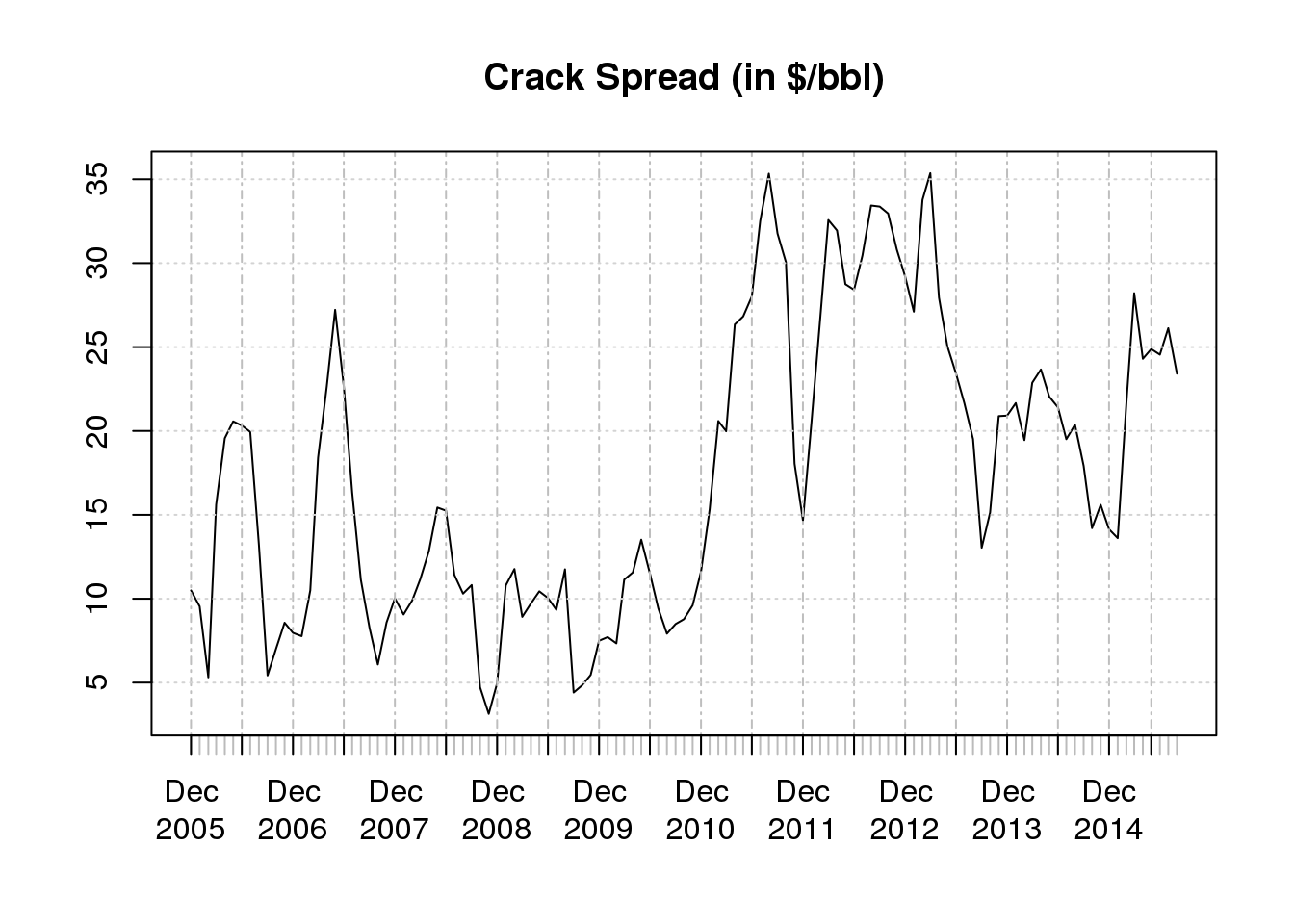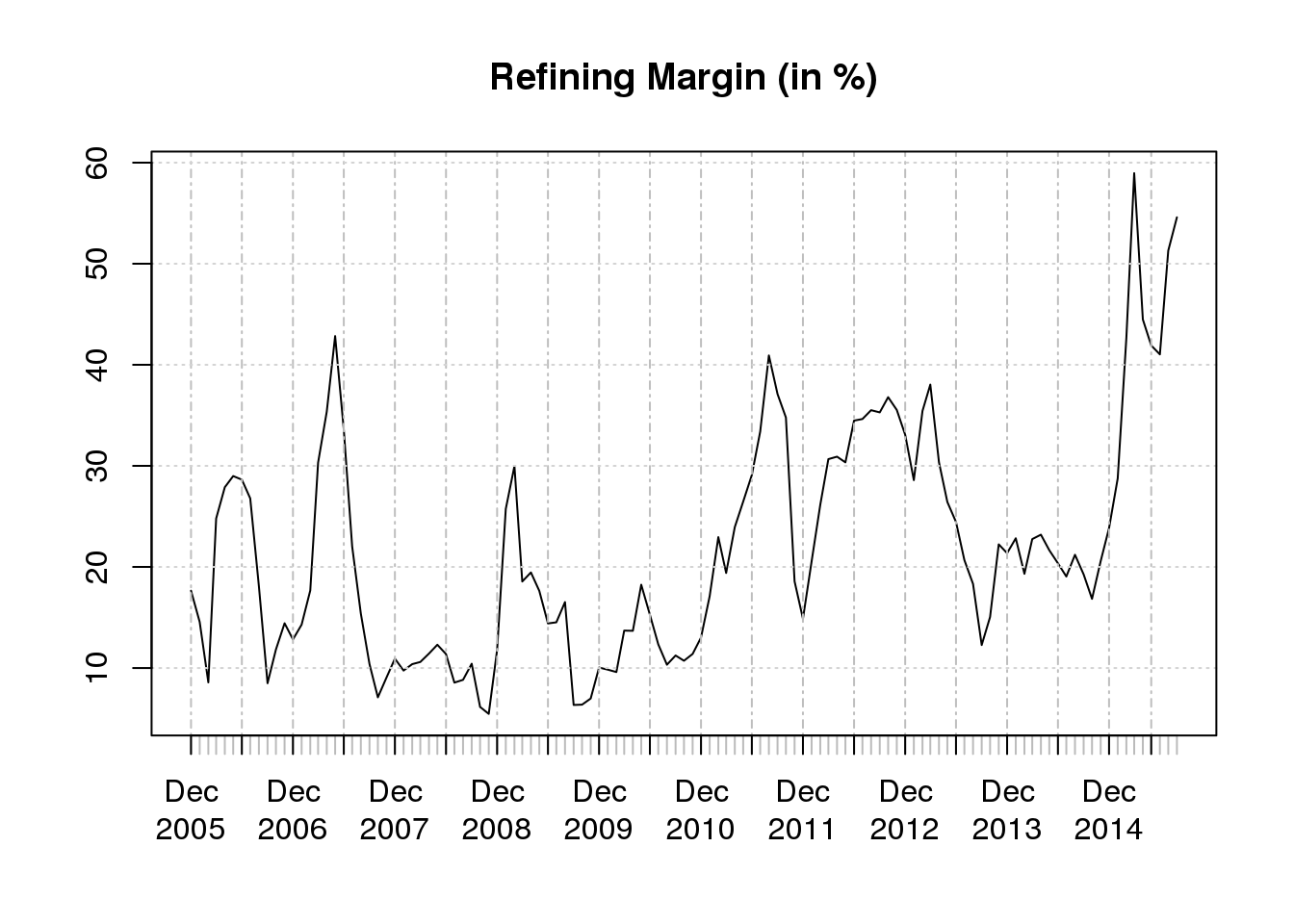The following will download monthly NYMEX CL, RB, and HO from the EIA, and calculate a monthly 3:2:1 crack spread (in $/bbl) and gross refining margin. RB and HO are multiplied by 42 in the crack spread calculation because there are 42 gallons per barrel. Note RB and HO are quoted per gallon, and CL is quoted per barrel. RB is multiplied by 2/3, and HO is multiplied by 1/3 to state the resulting crack spread as per barrel. The spread is 3 barrels of CL, for 2 barrels of RB and 1 barrel of HO. This is equivalently 1 barrel of crude for 2/3 barrel of RB and 1/3 barrel of HO. library(EIAdata) ## load my EIA API key. You'll need one also. key <- source('~/eia_key')$value

## get data
oil <- getEIA(key = key, ID = 'PET.RCLC1.M')
rbob <- getEIA(key = key, ID = 'PET.EER_EPMRR_PE1_Y35NY_DPG.M')
ho <- getEIA(key = key, ID = 'PET.EER_EPD2F_PE1_Y35NY_DPG.M')

## merge to common time interval
data <- merge.xts(oil, rbob, ho, join = 'inner')
names(data) <- c("oil", "rbob", "ho")

## calculate crack spread
crack <- data$rbob * 2/3 * 42 + data$ho * 1/3 * 42 - data$oil names(crack) <- c("crack spread") ## calculate gross refining margin refining.margin <- crack / (data$oil)

Time series plot of the crack spread.

## plot crack
plot(crack, main = "Crack Spread (in \$/bbl)", type = 'l')Time series plot of the refining margin. Can you explain the increase in the refining margin post 2008, and post 2014?

## plot refining margin
plot(refining.margin * 100, main = "Refining Margin (in %)", type = 'l')